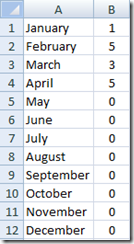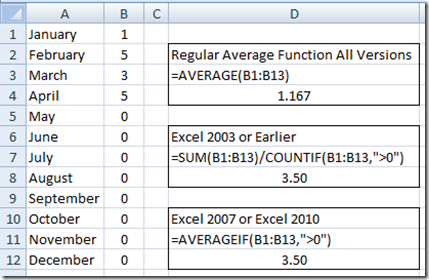# Excel Formulas: Average a Range and Ignore Zero’s (0 Value)Excel formulas are a wonderful thing, but they may not provide all the flexibility you want when calculating information for your Executive Dashboard display.  Sometimes you need to make your own formulas when Excel doesn’t provide a built-in function that gives you the desired outcome.  Now the wonderful Microsoft developers are making great strides to add new Excel Functions that will meet your needs, but what if the function doesn’t exist?

Perhaps you are working in an earlier version of Excel for a client and you need a function that is in 2010, but not 2003.  What do you do?  Well you can probably derive the value you need using a combination of other formulas.  One such Excel Function that does not exist in earlier versions is what we are going to talk about today.

Recently, I was working with a client using Excel 2003 and they were calculating an average of their data for the entire year, but when the data for future months shows up as a Zero, their averages were wrong.  So how can we ignore Zero’s in an average calculation.

Here is the sample data as it was only April so they only had 4 months of data:With the data above, when you calculate the average of B1:B4, you will get an answer of 1.167.  Not what we hoping to get.  How can we ignore the zero values?  Now when you use a standard Average formula, you have to do one of 2 things: 1) either change your average formula to only look at rows B1:B4 or you have to delete the cells that have a Zero entered.  Both of these approaches have their drawbacks.  Mostly this take time and may introduce errors into your spreadsheet.  Also, what happens if your data set is a lot greater than 12 rows?  You would have to spend lots of time deleting or replacing zeros and/or sorting the data and modifying your formulas.  There has to be a better way.  There is, but it depends on the version of Excel that you have.

Averaging Zero’s in Excel 2007 or Excel 2010 with AverageIF

Now the awesome Excel developers have created a formula for you if you are in Excel 2007 or newer.  The formula is called “AverageIF”.  It is really simple to use.  Here is how the Excel Function is set up:

=AVERAGEIF(Range,Criteria)

Range: First you type in = then AVERAGEIF( now you need to enter the range of data that you want to average by either highlighting the data or entering in the named range.

Criteria: Now for the criteria, there is just one catch.  If you want your criteria to have some logic that is not Equal to, then you will have to enter the criteria enclosed in quotes.

Here is a sample:   =AVERAGEIF(B1:B13,”>0″)

You will see that the criteria that will exclude Zeros from the average is entered in “quotes” as “>0”.

This formula will give you the desired calculation in Excel by excluding Zero’s from the Calculation.  On the sample of data above you will now get an average of 3.5 versus 1.167 when all the Zeros are included)

There, we did it, but what about my example where my client has Excel 2003?  AverageIF is not available in Excel 2003.  Can it be done with existing functions that do not require manual intervention?  Yes it can.

Averaging Zero’s in Excel 2003 with Sum and CountIF

So there is no AverageIF Function in Excel 2003, so what can we do?  We must combine two functions in the formula that will give us the desired calculation of averaging a range of numbers while ignoring zero’s.  Here is how the Excel Function is set up:

=SUM(B1:B13)/COUNTIF(B1:B13,”>0″)

What does it do?  It brings us all back to high school algebra and breaks down the average formula into its two components.  One, adding up all the values in the range (Sum in the numerator) and it divides that sum by the total number of items added in the sum that are greater than zero.  In the case of our data sample above, it will add up all the values in the range even if they are zero and gets the total of 14 then it divides 14 by the count of items that are greater than zero of 4 and gives us the average of 3.5.  The regular excel average function would give us 1.167 = 14/12.

# If you found the website and tutorials helpful, please consider donating to keep the lights on.

## Donate with PayPal here:So remember AverageIF function in Excel 2007 and Excel 2010, but if you are working in Excel 2003 you can always use Sum and CountIF.

I hope this helps you build the datasets for your next Excel company dashboard project!

Steve=True

1.steve

The AverageIF function as shown doesn’ work. Excel 2010 gives you a “#Value!” error when using the “>0” in the formula. This webpage should be removed from the internet as it is incorrect information.

•SteveEqualsTrue

Hi Heart4fun, sorry it isn’t working for you and that you think this page should be removed. I just tried it again and I am not getting a #value error. In a new example, I typed in a value of 1 in cells A1:A10 and a 0 in cell A11. Then in A12 I put in this formula: =AVERAGEIF(A1:A11,”>0″) and it returns a value of 1 (thus not putting the zero value into the average). What does your entire formula look like? Perhaps there is another issue that you are having. Hopefully I can help. Steve=True

•Pete

I just tried the same type of test and the AVERAGEIF and “>0” combination works just fine for me.
R/Pete

•SteveEqualsTrue

Thanks Pete. Much appreciated. Steve=True

• # If you found the website and tutorials helpful, please consider donating to keep the lights on.

## Donate with PayPal here:•Shane Andrews

Hi really need your help, I would like to know how I can average different cells in different columns and ignoring zeros. For e.g take columns a to k, a will have an average that i need then c will have an average that i need and then e etc. The other columns will have other figures which I do not need. Please help i have used the following but did not help, =AverageIF(c3,e3,g3,i3,k3,”>0″) I have tried messing about with this but not sure where I am going wrong your help will be appreciated, many thanks in advance